Скачать презентацию Inventory Control Model Kusdhianto Setiawan Gadjah Mada University

1b2e6478205b8927c2f82d00790d5149.ppt

• Количество слайдов: 26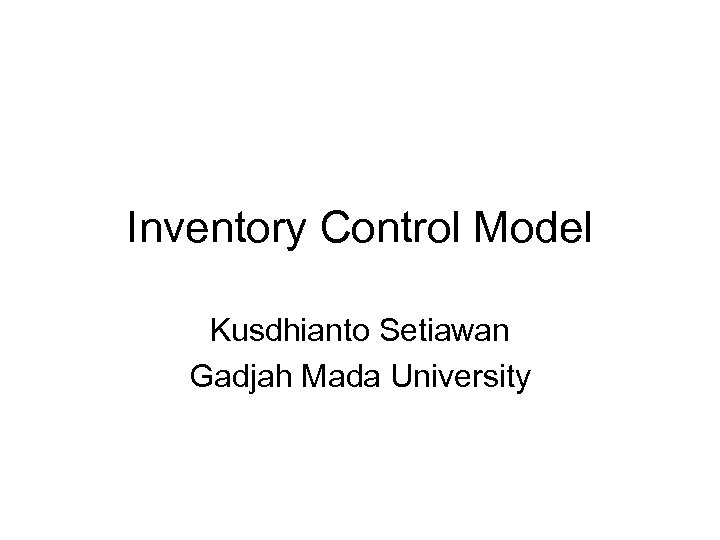Inventory Control Model Kusdhianto Setiawan Gadjah Mada University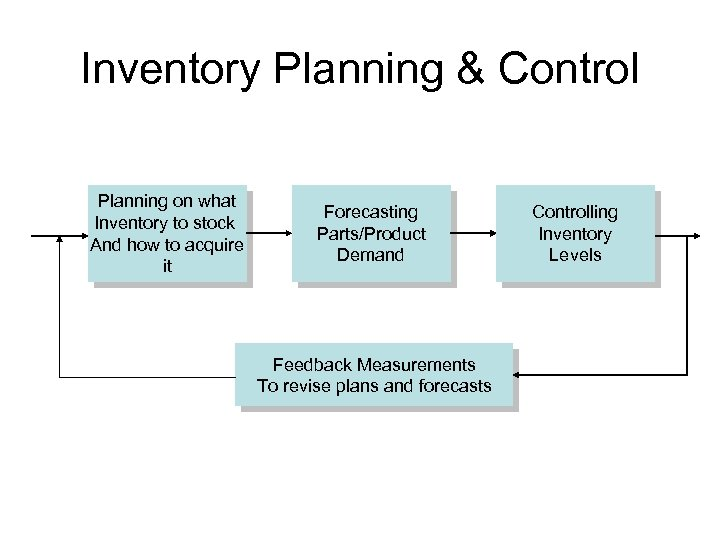Inventory Planning & Control Planning on what Inventory to stock And how to acquire it Forecasting Parts/Product Demand Feedback Measurements To revise plans and forecasts Controlling Inventory Levels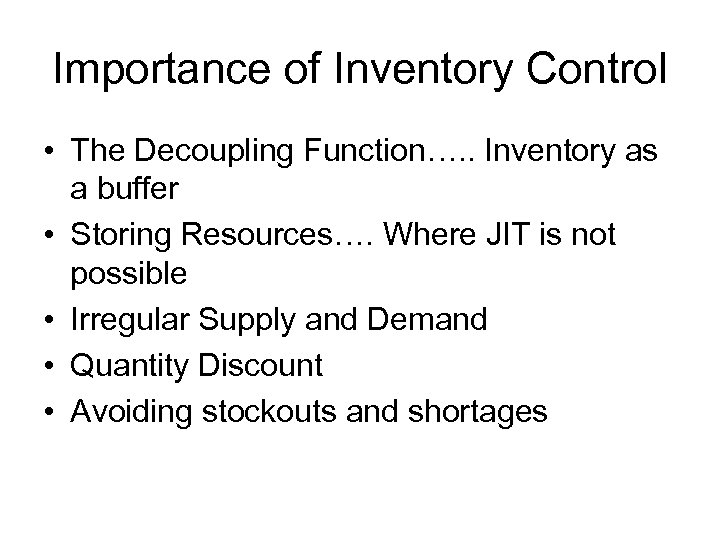Importance of Inventory Control • The Decoupling Function…. . Inventory as a buffer • Storing Resources…. Where JIT is not possible • Irregular Supply and Demand • Quantity Discount • Avoiding stockouts and shortages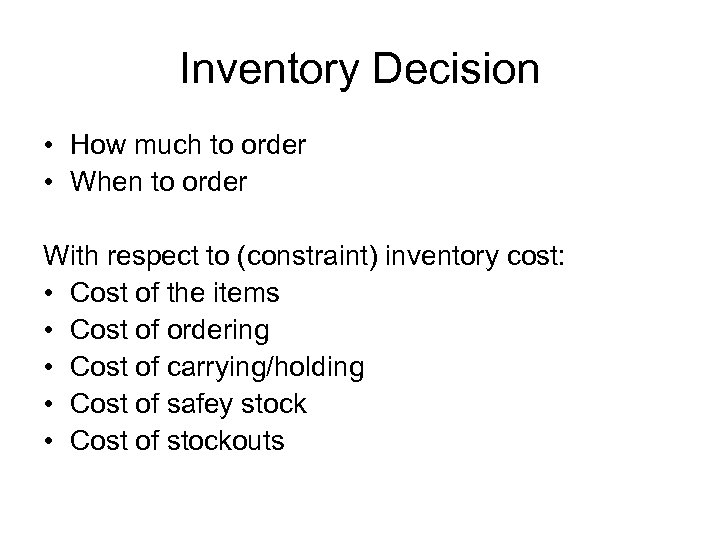Inventory Decision • How much to order • When to order With respect to (constraint) inventory cost: • Cost of the items • Cost of ordering • Cost of carrying/holding • Cost of safey stock • Cost of stockouts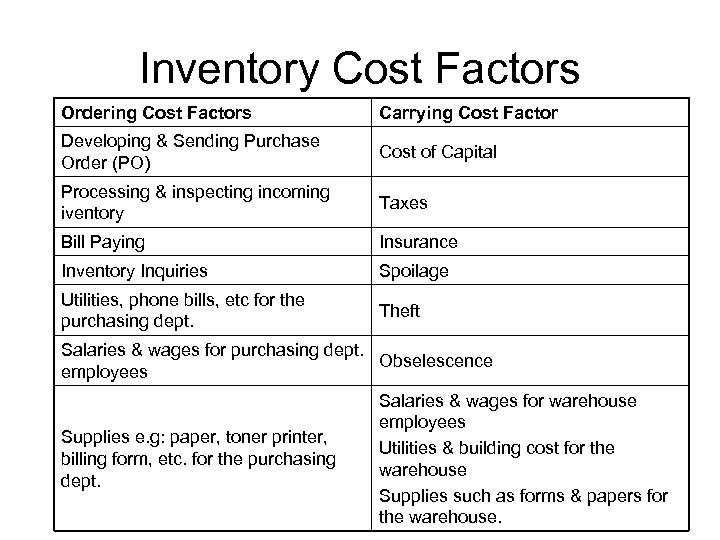Inventory Cost Factors Ordering Cost Factors Carrying Cost Factor Developing & Sending Purchase Order (PO) Cost of Capital Processing & inspecting incoming iventory Taxes Bill Paying Insurance Inventory Inquiries Spoilage Utilities, phone bills, etc for the purchasing dept. Theft Salaries & wages for purchasing dept. Obselescence employees Supplies e. g: paper, toner printer, billing form, etc. for the purchasing dept. Salaries & wages for warehouse employees Utilities & building cost for the warehouse Supplies such as forms & papers for the warehouse.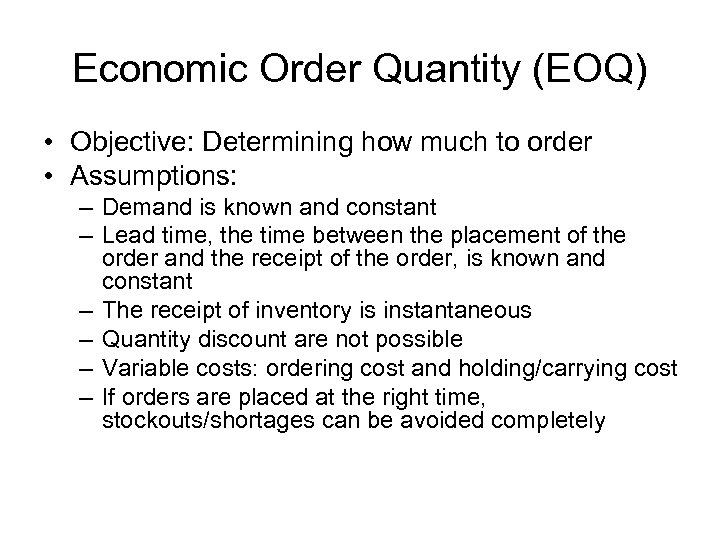Economic Order Quantity (EOQ) • Objective: Determining how much to order • Assumptions: – Demand is known and constant – Lead time, the time between the placement of the order and the receipt of the order, is known and constant – The receipt of inventory is instantaneous – Quantity discount are not possible – Variable costs: ordering cost and holding/carrying cost – If orders are placed at the right time, stockouts/shortages can be avoided completely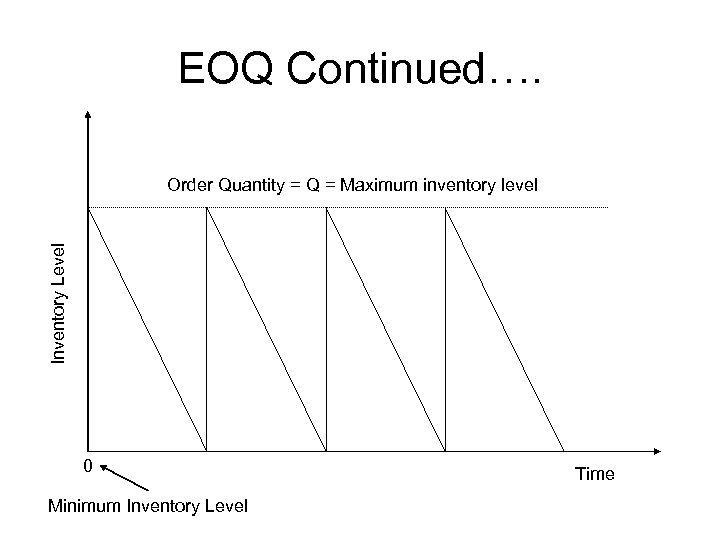EOQ Continued…. Inventory Level Order Quantity = Q = Maximum inventory level 0 Minimum Inventory Level Time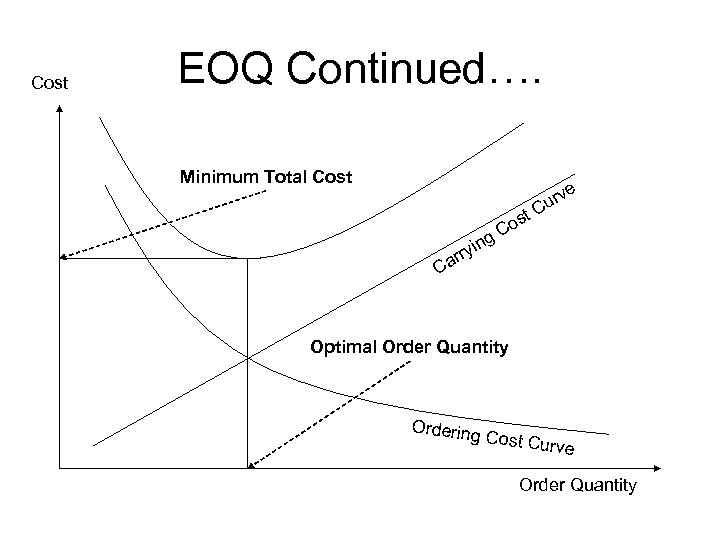Cost EOQ Continued…. Minimum Total Cost ng ryi ar t os C e urv C C Optimal Order Quantity Ordering Cost Cu rv e Order Quantity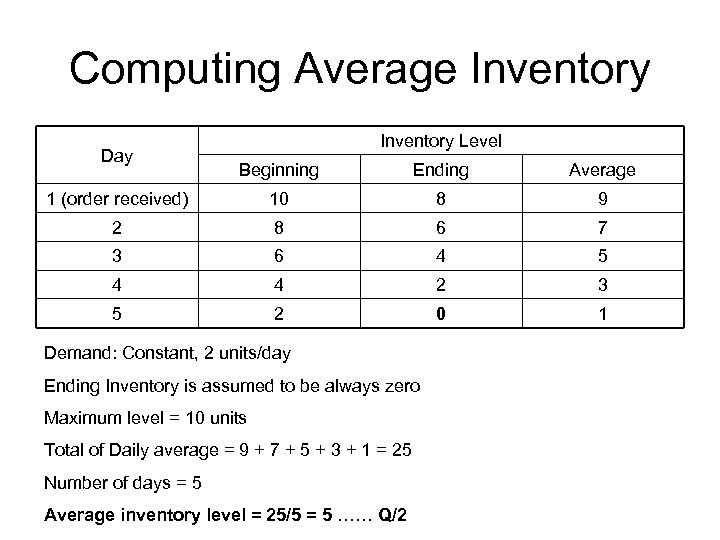Computing Average Inventory Day Inventory Level Beginning Ending Average 1 (order received) 10 8 9 2 8 6 7 3 6 4 5 4 4 2 3 5 2 0 1 Demand: Constant, 2 units/day Ending Inventory is assumed to be always zero Maximum level = 10 units Total of Daily average = 9 + 7 + 5 + 3 + 1 = 25 Number of days = 5 Average inventory level = 25/5 = 5 …… Q/2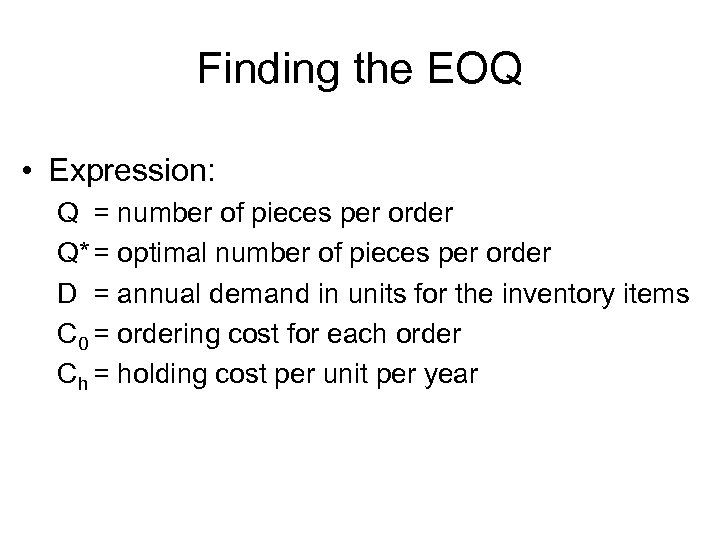Finding the EOQ • Expression: Q = number of pieces per order Q* = optimal number of pieces per order D = annual demand in units for the inventory items C 0 = ordering cost for each order Ch = holding cost per unit per year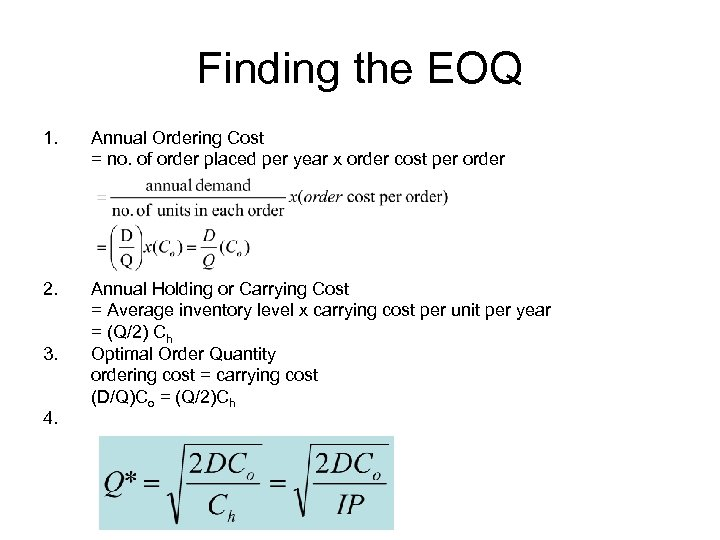Finding the EOQ 1. Annual Ordering Cost = no. of order placed per year x order cost per order 2. Annual Holding or Carrying Cost = Average inventory level x carrying cost per unit per year = (Q/2) Ch Optimal Order Quantity ordering cost = carrying cost (D/Q)Co = (Q/2)Ch 3. 4.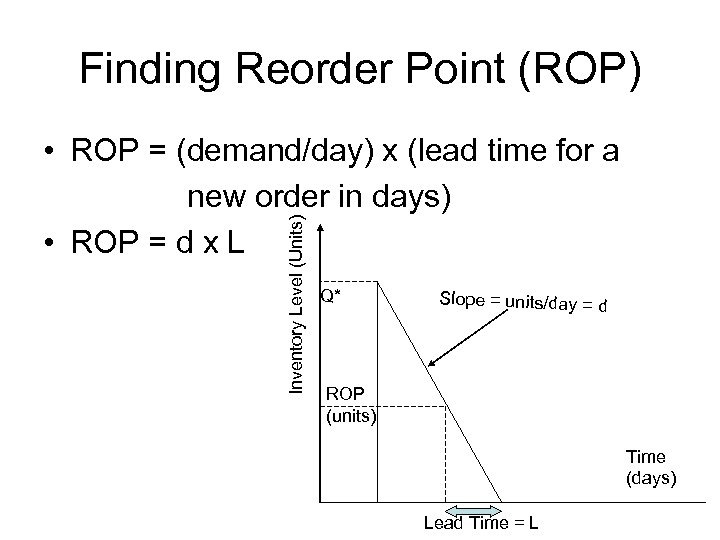Finding Reorder Point (ROP) Inventory Level (Units) • ROP = (demand/day) x (lead time for a new order in days) • ROP = d x L Q* Slope = units/day = d ROP (units) Time (days) Lead Time = L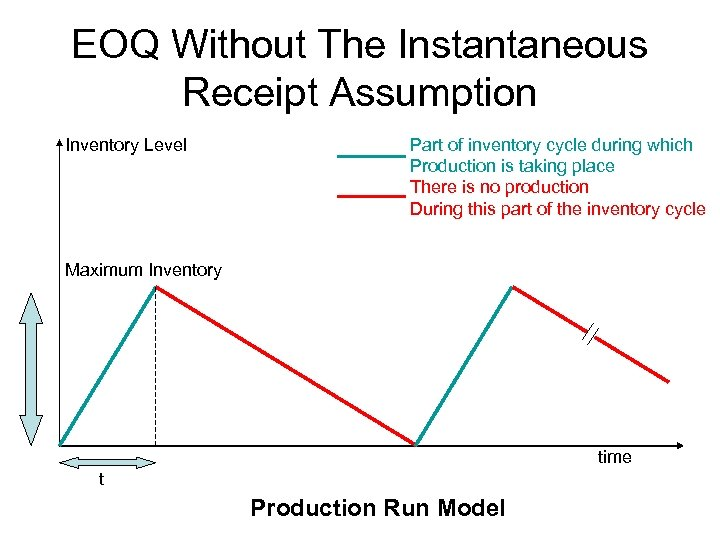EOQ Without The Instantaneous Receipt Assumption Inventory Level Part of inventory cycle during which Production is taking place There is no production During this part of the inventory cycle Maximum Inventory time t Production Run Model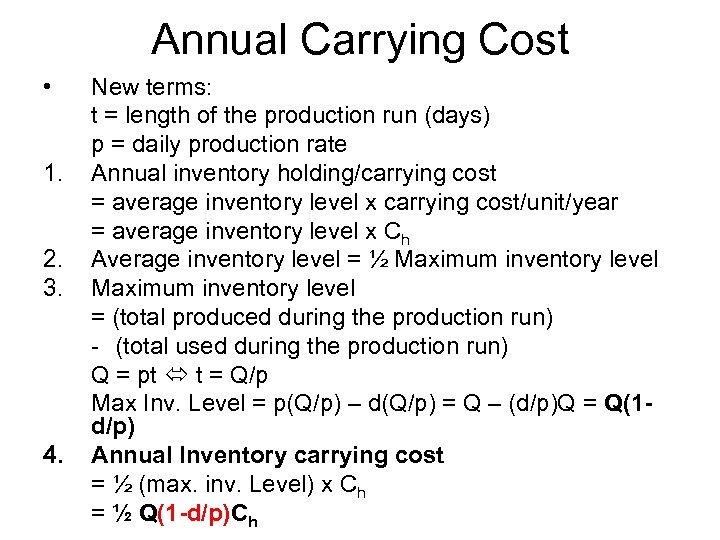Annual Carrying Cost • 1. 2. 3. 4. New terms: t = length of the production run (days) p = daily production rate Annual inventory holding/carrying cost = average inventory level x carrying cost/unit/year = average inventory level x Ch Average inventory level = ½ Maximum inventory level = (total produced during the production run) - (total used during the production run) Q = pt t = Q/p Max Inv. Level = p(Q/p) – d(Q/p) = Q – (d/p)Q = Q(1 d/p) Annual Inventory carrying cost = ½ (max. inv. Level) x Ch = ½ Q(1 -d/p)Ch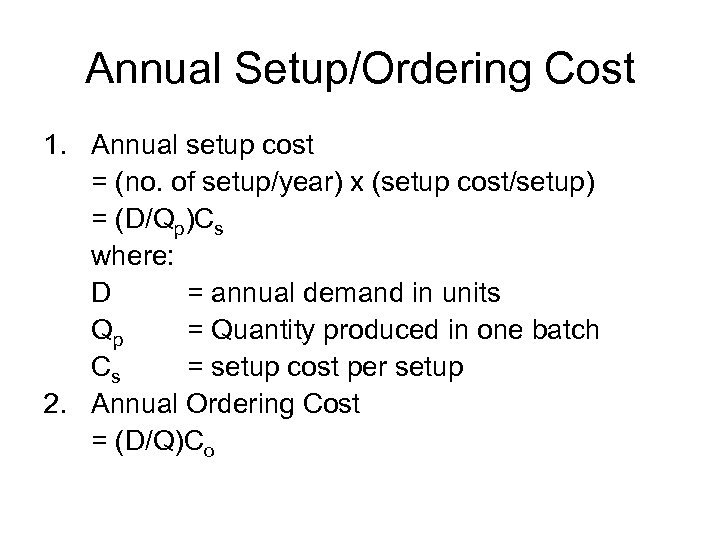Annual Setup/Ordering Cost 1. Annual setup cost = (no. of setup/year) x (setup cost/setup) = (D/Qp)Cs where: D = annual demand in units Qp = Quantity produced in one batch Cs = setup cost per setup 2. Annual Ordering Cost = (D/Q)Co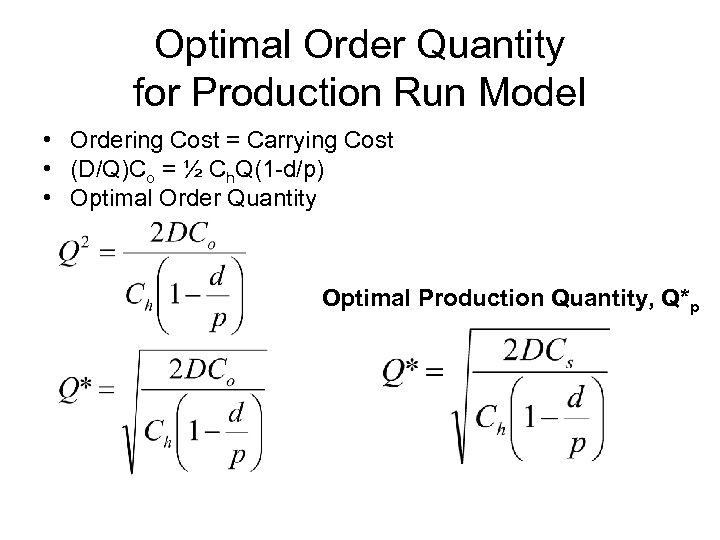Optimal Order Quantity for Production Run Model • Ordering Cost = Carrying Cost • (D/Q)Co = ½ Ch. Q(1 -d/p) • Optimal Order Quantity Optimal Production Quantity, Q*p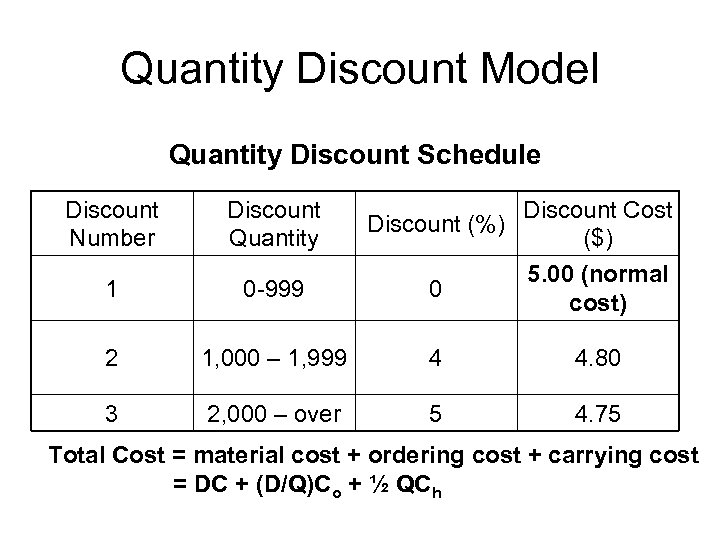Quantity Discount Model Quantity Discount Schedule Discount Number Discount Quantity Discount (%) Discount Cost (\$) 1 0 -999 0 5. 00 (normal cost) 2 1, 000 – 1, 999 4 4. 80 3 2, 000 – over 5 4. 75 Total Cost = material cost + ordering cost + carrying cost = DC + (D/Q)Co + ½ QCh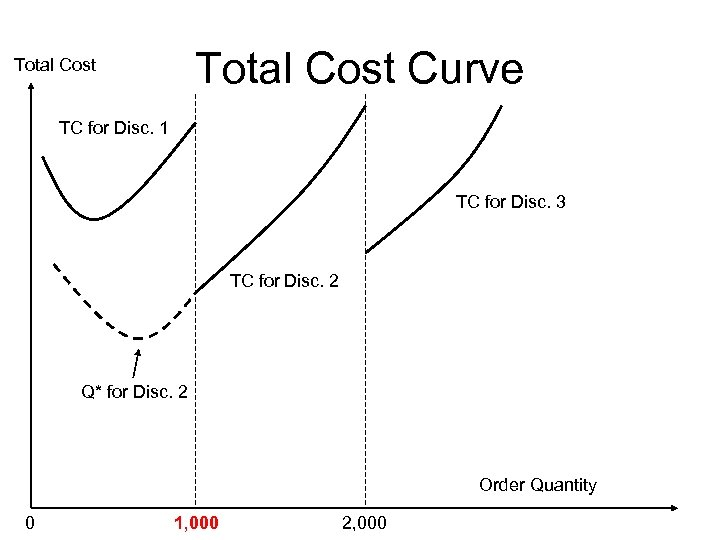Total Cost Curve Total Cost TC for Disc. 1 TC for Disc. 3 TC for Disc. 2 Q* for Disc. 2 Order Quantity 0 1, 000 2, 000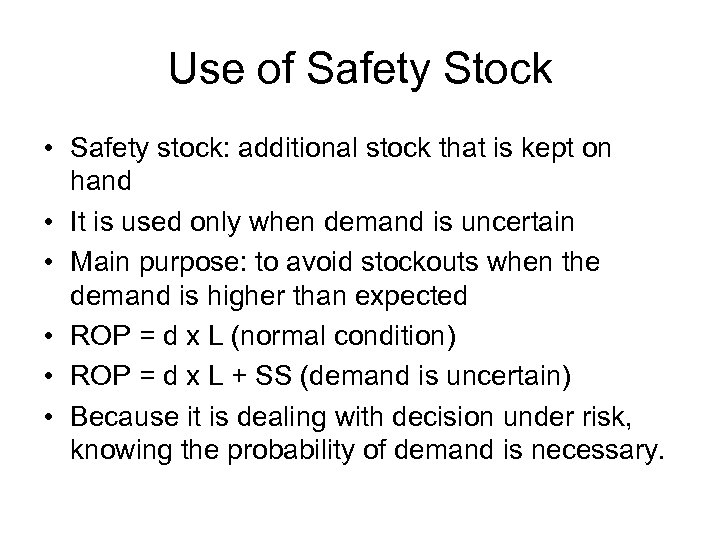Use of Safety Stock • Safety stock: additional stock that is kept on hand • It is used only when demand is uncertain • Main purpose: to avoid stockouts when the demand is higher than expected • ROP = d x L (normal condition) • ROP = d x L + SS (demand is uncertain) • Because it is dealing with decision under risk, knowing the probability of demand is necessary.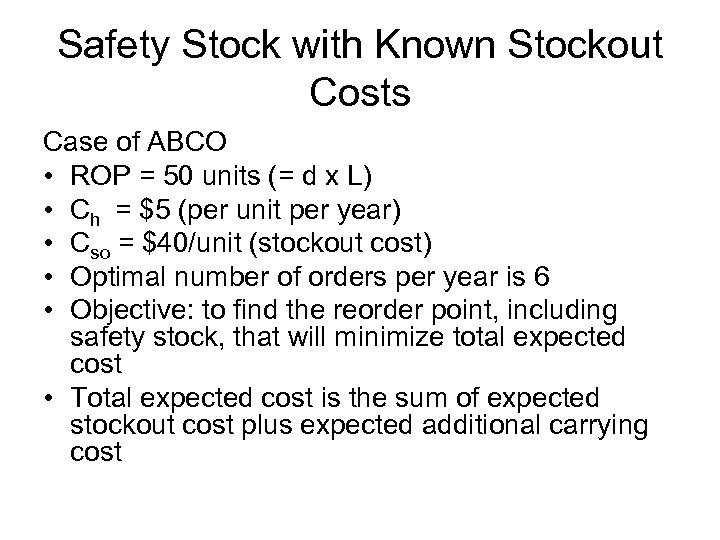Safety Stock with Known Stockout Costs Case of ABCO • ROP = 50 units (= d x L) • Ch = \$5 (per unit per year) • Cso = \$40/unit (stockout cost) • Optimal number of orders per year is 6 • Objective: to find the reorder point, including safety stock, that will minimize total expected cost • Total expected cost is the sum of expected stockout cost plus expected additional carrying cost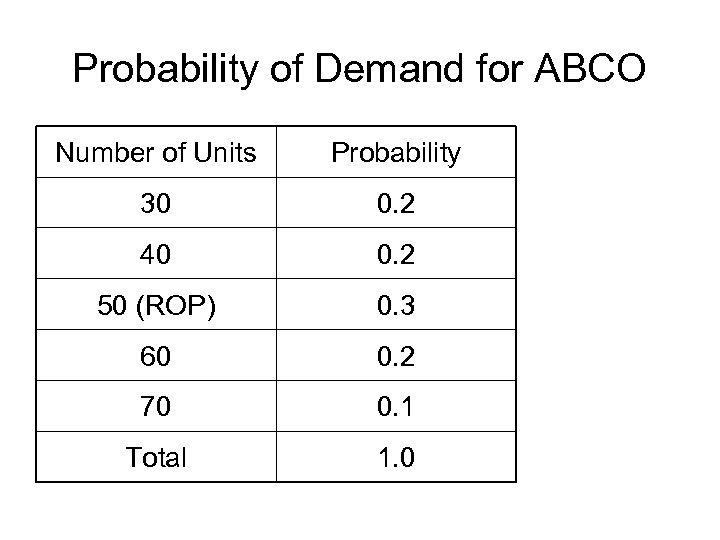Probability of Demand for ABCO Number of Units Probability 30 0. 2 40 0. 2 50 (ROP) 0. 3 60 0. 2 70 0. 1 Total 1. 0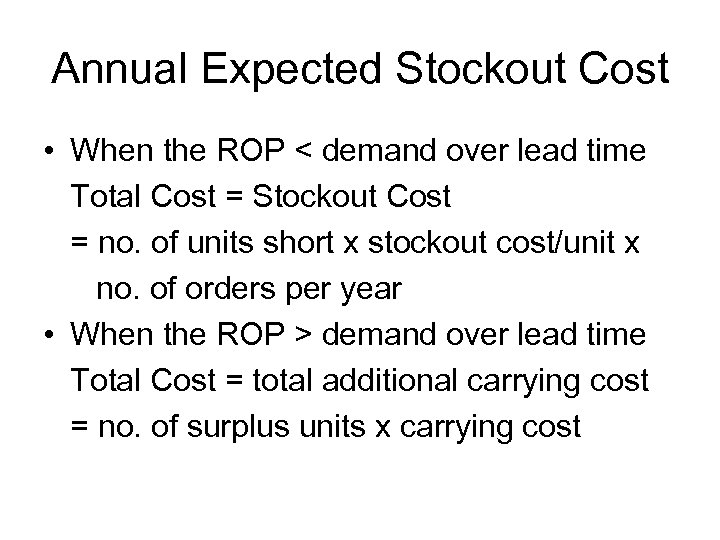Annual Expected Stockout Cost • When the ROP < demand over lead time Total Cost = Stockout Cost = no. of units short x stockout cost/unit x no. of orders per year • When the ROP > demand over lead time Total Cost = total additional carrying cost = no. of surplus units x carrying cost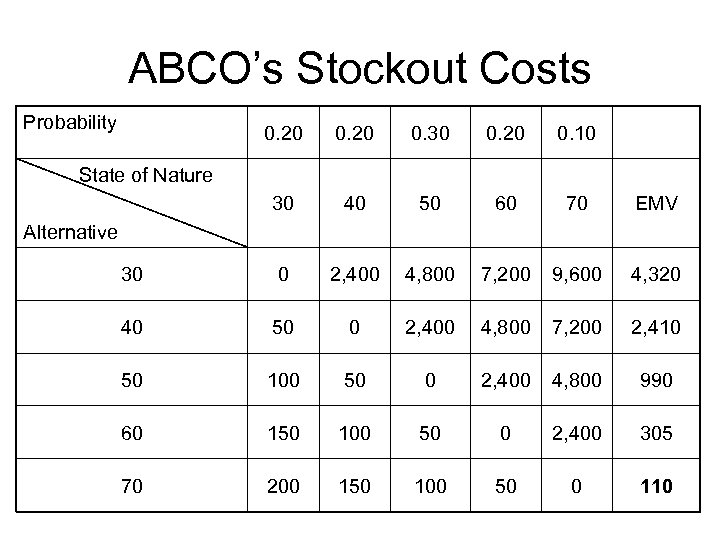ABCO’s Stockout Costs Probability 0. 20 0. 30 0. 20 0. 10 30 40 50 60 70 EMV 30 0 2, 400 4, 800 7, 200 9, 600 4, 320 40 50 0 2, 400 4, 800 7, 200 2, 410 50 100 50 0 2, 400 4, 800 990 60 150 100 50 0 2, 400 305 70 200 150 100 50 0 110 State of Nature Alternative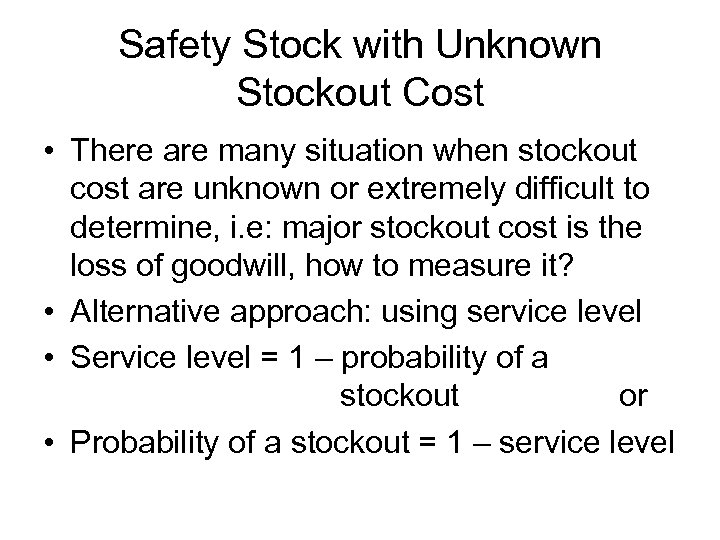Safety Stock with Unknown Stockout Cost • There are many situation when stockout cost are unknown or extremely difficult to determine, i. e: major stockout cost is the loss of goodwill, how to measure it? • Alternative approach: using service level • Service level = 1 – probability of a stockout or • Probability of a stockout = 1 – service level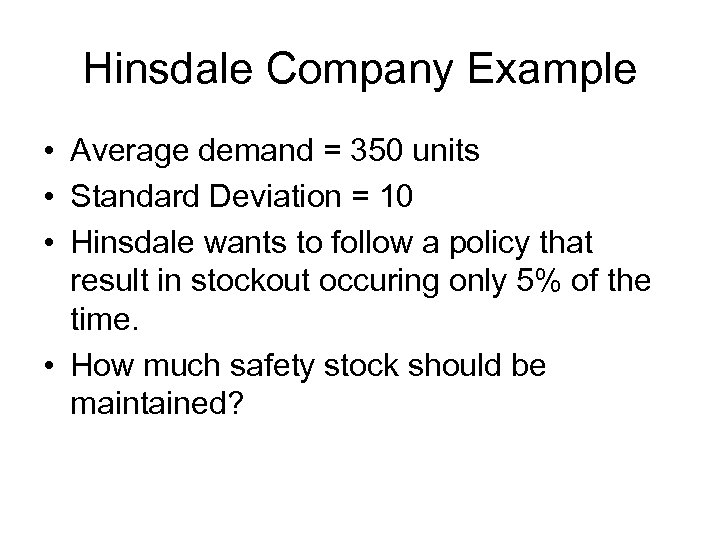Hinsdale Company Example • Average demand = 350 units • Standard Deviation = 10 • Hinsdale wants to follow a policy that result in stockout occuring only 5% of the time. • How much safety stock should be maintained?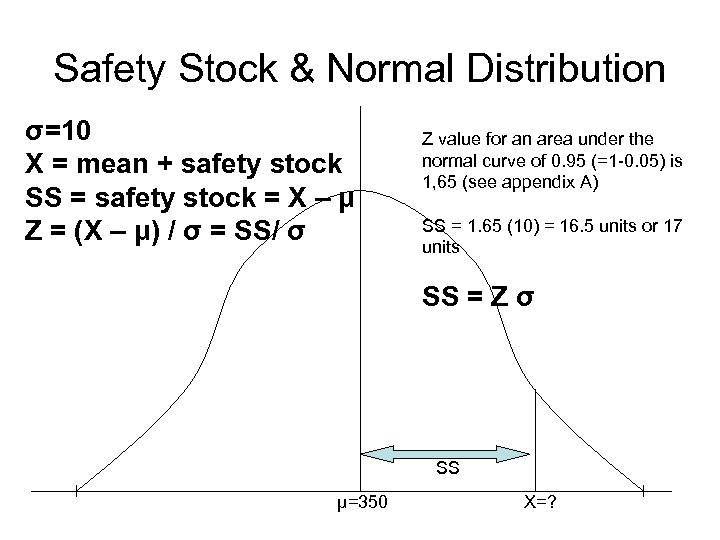Safety Stock & Normal Distribution σ=10 X = mean + safety stock SS = safety stock = X – μ Z = (X – μ) / σ = SS/ σ Z value for an area under the normal curve of 0. 95 (=1 -0. 05) is 1, 65 (see appendix A) SS = 1. 65 (10) = 16. 5 units or 17 units SS = Z σ SS μ=350 X=?